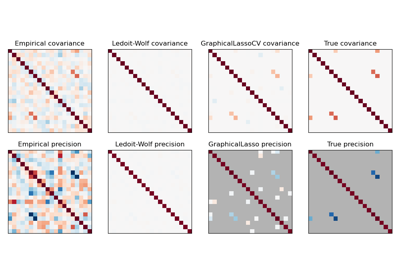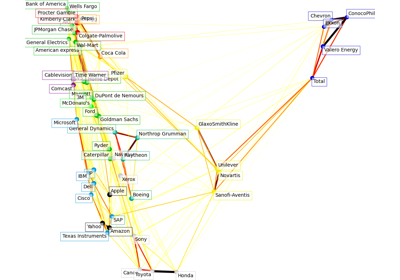# sklearn.covariance.GraphicalLassoCV¶

class sklearn.covariance.GraphicalLassoCV(*, alphas=4, n_refinements=4, cv=None, tol=0.0001, enet_tol=0.0001, max_iter=100, mode='cd', n_jobs=None, verbose=False, assume_centered=False)[source]

Sparse inverse covariance w/ cross-validated choice of the l1 penalty.

See glossary entry for cross-validation estimator.

Read more in the User Guide.

Changed in version v0.20: GraphLassoCV has been renamed to GraphicalLassoCV

Parameters
alphasint or array-like of shape (n_alphas,), dtype=float, default=4

If an integer is given, it fixes the number of points on the grids of alpha to be used. If a list is given, it gives the grid to be used. See the notes in the class docstring for more details. Range is (0, inf] when floats given.

n_refinementsint, default=4

The number of times the grid is refined. Not used if explicit values of alphas are passed. Range is [1, inf).

cvint, cross-validation generator or iterable, default=None

Determines the cross-validation splitting strategy. Possible inputs for cv are:

• None, to use the default 5-fold cross-validation,

• integer, to specify the number of folds.

• An iterable yielding (train, test) splits as arrays of indices.

For integer/None inputs KFold is used.

Refer User Guide for the various cross-validation strategies that can be used here.

Changed in version 0.20: cv default value if None changed from 3-fold to 5-fold.

tolfloat, default=1e-4

The tolerance to declare convergence: if the dual gap goes below this value, iterations are stopped. Range is (0, inf].

enet_tolfloat, default=1e-4

The tolerance for the elastic net solver used to calculate the descent direction. This parameter controls the accuracy of the search direction for a given column update, not of the overall parameter estimate. Only used for mode=’cd’. Range is (0, inf].

max_iterint, default=100

Maximum number of iterations.

mode{‘cd’, ‘lars’}, default=’cd’

The Lasso solver to use: coordinate descent or LARS. Use LARS for very sparse underlying graphs, where number of features is greater than number of samples. Elsewhere prefer cd which is more numerically stable.

n_jobsint, default=None

number of jobs to run in parallel. None means 1 unless in a joblib.parallel_backend context. -1 means using all processors. See Glossary for more details.

Changed in version v0.20: n_jobs default changed from 1 to None

verbosebool, default=False

If verbose is True, the objective function and duality gap are printed at each iteration.

assume_centeredbool, default=False

If True, data are not centered before computation. Useful when working with data whose mean is almost, but not exactly zero. If False, data are centered before computation.

Attributes
location_ndarray of shape (n_features,)

Estimated location, i.e. the estimated mean.

covariance_ndarray of shape (n_features, n_features)

Estimated covariance matrix.

precision_ndarray of shape (n_features, n_features)

Estimated precision matrix (inverse covariance).

alpha_float

Penalization parameter selected.

cv_alphas_list of shape (n_alphas,), dtype=float

All penalization parameters explored.

grid_scores_ndarray of shape (n_alphas, n_folds)

Log-likelihood score on left-out data across folds.

n_iter_int

Number of iterations run for the optimal alpha.

Notes

The search for the optimal penalization parameter (alpha) is done on an iteratively refined grid: first the cross-validated scores on a grid are computed, then a new refined grid is centered around the maximum, and so on.

One of the challenges which is faced here is that the solvers can fail to converge to a well-conditioned estimate. The corresponding values of alpha then come out as missing values, but the optimum may be close to these missing values.

Examples

>>> import numpy as np
>>> from sklearn.covariance import GraphicalLassoCV
>>> true_cov = np.array([[0.8, 0.0, 0.2, 0.0],
...                      [0.0, 0.4, 0.0, 0.0],
...                      [0.2, 0.0, 0.3, 0.1],
...                      [0.0, 0.0, 0.1, 0.7]])
>>> np.random.seed(0)
>>> X = np.random.multivariate_normal(mean=[0, 0, 0, 0],
...                                   cov=true_cov,
...                                   size=200)
>>> cov = GraphicalLassoCV().fit(X)
>>> np.around(cov.covariance_, decimals=3)
array([[0.816, 0.051, 0.22 , 0.017],
[0.051, 0.364, 0.018, 0.036],
[0.22 , 0.018, 0.322, 0.094],
[0.017, 0.036, 0.094, 0.69 ]])
>>> np.around(cov.location_, decimals=3)
array([0.073, 0.04 , 0.038, 0.143])


Methods

 error_norm(comp_cov[, norm, scaling, squared]) Computes the Mean Squared Error between two covariance estimators. fit(X[, y]) Fits the GraphicalLasso covariance model to X. get_params([deep]) Get parameters for this estimator. Getter for the precision matrix. Computes the squared Mahalanobis distances of given observations. score(X_test[, y]) Computes the log-likelihood of a Gaussian data set with self.covariance_ as an estimator of its covariance matrix. set_params(**params) Set the parameters of this estimator.
__init__(*, alphas=4, n_refinements=4, cv=None, tol=0.0001, enet_tol=0.0001, max_iter=100, mode='cd', n_jobs=None, verbose=False, assume_centered=False)[source]

Initialize self. See help(type(self)) for accurate signature.

error_norm(comp_cov, norm='frobenius', scaling=True, squared=True)[source]

Computes the Mean Squared Error between two covariance estimators. (In the sense of the Frobenius norm).

Parameters
comp_covarray-like of shape (n_features, n_features)

The covariance to compare with.

norm{“frobenius”, “spectral”}, default=”frobenius”

The type of norm used to compute the error. Available error types: - ‘frobenius’ (default): sqrt(tr(A^t.A)) - ‘spectral’: sqrt(max(eigenvalues(A^t.A)) where A is the error (comp_cov - self.covariance_).

scalingbool, default=True

If True (default), the squared error norm is divided by n_features. If False, the squared error norm is not rescaled.

squaredbool, default=True

Whether to compute the squared error norm or the error norm. If True (default), the squared error norm is returned. If False, the error norm is returned.

Returns
resultfloat

The Mean Squared Error (in the sense of the Frobenius norm) between self and comp_cov covariance estimators.

fit(X, y=None)[source]

Fits the GraphicalLasso covariance model to X.

Parameters
Xarray-like of shape (n_samples, n_features)

Data from which to compute the covariance estimate

yIgnored

Not used, present for API consistence purpose.

Returns
selfobject
get_params(deep=True)[source]

Get parameters for this estimator.

Parameters
deepbool, default=True

If True, will return the parameters for this estimator and contained subobjects that are estimators.

Returns
paramsmapping of string to any

Parameter names mapped to their values.

get_precision()[source]

Getter for the precision matrix.

Returns
precision_array-like of shape (n_features, n_features)

The precision matrix associated to the current covariance object.

mahalanobis(X)[source]

Computes the squared Mahalanobis distances of given observations.

Parameters
Xarray-like of shape (n_samples, n_features)

The observations, the Mahalanobis distances of the which we compute. Observations are assumed to be drawn from the same distribution than the data used in fit.

Returns
distndarray of shape (n_samples,)

Squared Mahalanobis distances of the observations.

score(X_test, y=None)[source]

Computes the log-likelihood of a Gaussian data set with self.covariance_ as an estimator of its covariance matrix.

Parameters
X_testarray-like of shape (n_samples, n_features)

Test data of which we compute the likelihood, where n_samples is the number of samples and n_features is the number of features. X_test is assumed to be drawn from the same distribution than the data used in fit (including centering).

yIgnored

Not used, present for API consistence purpose.

Returns
resfloat

The likelihood of the data set with self.covariance_ as an estimator of its covariance matrix.

set_params(**params)[source]

Set the parameters of this estimator.

The method works on simple estimators as well as on nested objects (such as pipelines). The latter have parameters of the form <component>__<parameter> so that it’s possible to update each component of a nested object.

Parameters
**paramsdict

Estimator parameters.

Returns
selfobject

Estimator instance.

## Examples using sklearn.covariance.GraphicalLassoCV¶